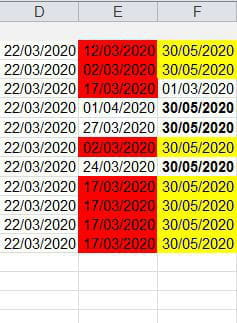# VBA: Color cells in table based on multiple condition

Solved/Closed
Posts
72
Registration date
Thursday July 18, 2019
Status
Member
Last seen
November 3, 2021
-
Posts
72
Registration date
Thursday July 18, 2019
Status
Member
Last seen
November 3, 2021
-
hello
i do code by loop but it doesn't completely work i appreciate if somebody help me why it occurred and give me the alternative ok let's get in subject i have sheet contains data all of them are dates in columns d,e,f when i run the code for column e it really works but in column f not exactly it should colored the cells are bold in column f with yellow and cells f4 should colored with red based on my code i hope somebody find me the explanation why happened
`Sub dat()Dim lr As Integer, i As Integer    lr = Cells(Rows.Count, "d").End(xlUp).Row    For i = 2 To lr        If Cells(i, 5) < Cells(i, 4) Then            Cells(i, 5).Interior.Color = vbRed            If Cells(i, 6) = Cells(i, 4) + 69 Then                Cells(i, 6).Interior.Color = vbYellow                If Month(CDate(Cells(i, 6))) = Month(Date) Then _                    Cells(i, 6).Interior.Color = vbRed            End If        End If    Next iEnd Sub`Related:

Posts
2829
Registration date
Sunday September 12, 2010
Status
Moderator
Last seen
November 22, 2021
490
Hi Abdel,

That is because of how you structured your IF statements.

5th code line:
If Cells(i, 5) < Cells(i, 4) Then
When this condition is not met, then the code skips to code line 12, thus skipping the other IF statements.

Here is your code, where each IF statement is handled one after the other:
```Sub dat()
Dim lr As Integer, i As Integer
lr = Cells(Rows.Count, "d").End(xlUp).Row
For i = 2 To lr
If Cells(i, 5) < Cells(i, 4) Then Cells(i, 5).Interior.Color = vbRed
If Cells(i, 6) = Cells(i, 4) + 69 Then Cells(i, 6).Interior.Color = vbYellow
If Month(CDate(Cells(i, 6))) = Month(Date) Then Cells(i, 6).Interior.Color = vbRed
Next i
End Sub```

The best way to check what is going on with your code is by placing your cursor somewhere in your code and then press F8 to go by each code line. By pressing F8 multiple times you can check where in your code it deviates from what you had in mind.

Best regards,
Trowa
Posts
72
Registration date
Thursday July 18, 2019
Status
Member
Last seen
November 3, 2021

thanks so much for this explanation and adjusting